### 散点分 [问题点数：300分，结帖人wufeng4552]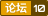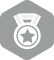2008年10月 总版技术专家分月排行榜第二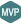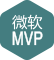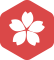2008年10月 MS-SQL Server大版内专家分月排行榜第一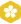2009年1月 MS-SQL Server大版内专家分月排行榜第二
2008年12月 MS-SQL Server大版内专家分月排行榜第二
2008年9月 MS-SQL Server大版内专家分月排行榜第二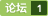echarts省份标注加散点效果

echarts实现双Y轴之散点和折线图

Matlab——离散点的随机区域分配
Matlab——离散点的随机区域分配nnnn 假设待定区域现在有200个离散点，我们随机挑选出10个离散点，并以这10个点为中心画半径为R（任意取）的圆。我们知道这10个圆在没有任何交集的情况下，共有1...

matlab三维散点的曲面画图
matlab三维散点的曲面画图，知道空间点x,y,z的坐标,大概有一万多个点.用MATLAB怎么画出三维图?有轮廓的那种,不是用plot3直接把点连起来的那种.好象没有直接的命令,可能要经过一些处理,有谁知道怎么做啊?请看程序吧，这里有
echarts地图上设置散点
n n n nnnnnnn前段时间，负责的项目上有一个开发 echarts 地图需求，有了之前的经验，配置地图相对来说简单很多。n但是这次要求在地图上铺设散点，这个是之前没有做过的，单独开发地图或者单独开发散点图难度都不是很大，但两种图形结合起来，还需要自定义，这就需要研究一下了。n通过这篇文章你可以学会如何在地图上自定义显示散点。n1、初始化地图n这里地图以广州市地...
3D散点 表面，2D散点，边界。 matlab 儿子的papa
clear allnclcnclfnn%% outlinen% find the out surface of vdw pointsnn%% mainn% % % load position of LYSn% % pos=load('../data/LYS/LYS_xyz');n% % n% % n_at=length(pos(:,1));n% % n% % tm=load('../data/LY
echars地图三级下钻+散点信息

Matlab--三维散点插值成曲面

echarts地图下钻+散点图

n n n 本来要做三维插值的，类似于等高线之类的，但是不会做，只做出来了三维散点图nnnnnnPaste_Image.pngnnnimport matplotlib.pyplot as pltnfrom mpl_toolkits.mplot3d import Axes3Dnfrom numpy import interpn#import scipy.interpol...
Python绘制散点对比图

matlab源码--散点数据生成格网、等高线并三维显示(通过克吕金插值)
kriging程序，用于散点数据生成格网，且可以绘制等高线图，二维或者三维显示。内有matlab的程序代码以及原理讲解的PDF

matplotlib绘制散点图详解（附代码）

ArcGIS加载天地图之散点聚合

Matplotlib学习笔记——简易散点图

matplotlib使用scatter画简单的散点图

import numpy as npnfrom matplotlib import pyplot as pltnimport mathnn=1024nx=np.random.normal(0,3,n) #产生高斯（正态）随机数，0为均值，3为方差，n表示sizeny=np.random.normal(0,2,n)nprint(x,y)nplt.xlabel('x') #设置...
echarts中国地图散点涟漪效果
echarts中国地图例子：http://gallery.echartsjs.com/editor.html?c=effectScatter-mapnnnvar data = [{n name: '郑州',n value: 100 //扩散的大小n },n {n name: '北京',n value: 100n },n ...
matlab 散点插值
matlab 空间散点 插值绘制曲面源代码
matplotlib画散点图
nimport matplotlib.pyplot as pltnimport numpy as npnnheight = [161,170, 182, 175, 173, 165]nweight = [50, 58, 80, 70, 69, 65]nnplt.scatter(height, weight)nnplt.show()nn#散点图 随机的，无相关性nN = 1000nx=np.rand...
MATLAB三维离散散点插值绘制三维曲面
MATLAB三维离散散点插值绘制三维曲面，给定X、Y、Z三维离散坐标点的值，结合插值算法，实现离散点插值，在MATLAB环境下实现三维曲面绘制
ECharts绘制地图分布，可自定义城市散点
&amp;lt;!DOCTYPE html&amp;gt;n&amp;lt;html&amp;gt;n &amp;lt;head&amp;gt;n &amp;lt;meta charset=&quot;UTF-8&quot;&amp;gt;n &amp;lt;title&amp;gt;作业运行&amp;lt;/title&amp;gt;n &amp;lt;script src=&quot;js/echarts.min.js&quot;&amp;gt;&amp;
matlab绘制散点图——指定散点落于一个圆形区域内
matlab绘制散点图——指定散点落于一个圆形区域内nnnn问题：nn在做海豚捕食沙丁鱼的仿真时，我们将沙丁鱼群看成了一个圆形区域内的散点，所以需要用matlab在一个圆形区域内绘制均匀分布的散点。nnmatlab代码实现nn% 海豚进入前沙丁鱼群的初始状态nN=200;%沙丁鱼群中沙丁鱼的数量n% 沙丁鱼群圆形区域的圆心nx0=-100;ny0=-100;n% 沙丁鱼群圆形区域的实际半径nR=1...
Python_简单交互作用回归分析及散点图矩阵
1、数据准备nimport urllib.requestnimport matplotlib.pyplot as pltnplt.style.use('ggplot')nimport pandas as pdn# 下载同时显示进度条ndef jdt(down, pic, all):n &quot;&quot;&quot;n @down : 已经下载的数据块n @pic : 数据块的大小n @all : ...

n n 例：加热一根钢管，因为热损耗及加热不完美均匀的问题，钢管上温度分布不均匀，通过测量不锈钢管上的温度分布，我们得到了下面这样的一个曲线，然后如何求钢管上的平均温度。nnnnnnn不锈钢管上温度分布nnn思路：这里我们用积分（曲线下面积）然后除以起始测量的距离差。直接求平均会有较大误差，一是温度分布没规律，二是如果测量时没有等距测量误差则更大nn方法一、Exc...
Matlab学习手记——Matlab三维散点插值成曲面

basemap生成地图散点图

echarts3d地图散点数据陷进地图的解决方案
echarts 3d地图数据散点下陷，下陷到地图里的数据是数据中的最小值，解决方法是，添加数据中不能出现的最小值，并且让它不现实出来，就可以解决想要数据下陷得问题

Echarts+百度地图_散点图完整版demo
echarts 引入百度地图api结合 ，echarts百度散点地图的demo
4.matplotlib绘制各种类型散点图标

&amp;gt; install.packages('GGally')n&amp;gt; library(GGally)n&amp;gt; theme_set(theme_minimal(20)) #设置主题n&amp;gt; set.seed(1836)n&amp;gt; pf_subset &amp;lt;- pf[,c(2,15)]n&amp;gt; names(pf_subset)n &quot;age&quot; &quot;www...

import numpy as npfrom scipy.interpolate import griddatapp=np.array((x.flatten(),y.flatten())).TValues=vf.flatten()Z0=griddata(pp,Values,np.array(xy))   x,y,vf为ndarray，xy为二维嵌套list，griddata默认为线性插值，还可选取...
echarts散点图，象限分析系列
nvar option = {n              title: [{n                text: '性格群体分析',n                subtext: '分析对象：XX区域',n                left: 'center',n                textStyle: {n                  fontSize: 1...
matlab求散点切线角度

matplotlib——散点图

Matlab给散点加上圆滑曲线
Matlab给散点加上圆滑曲线：nnnclc,clear,close allnx = [50,100,200,500,1000,2000,5000,10000,20000]ny = [2.64,2.21,1.38,0.564,0.263,0.396,1.07,1.98,3.14]nxx = 50:0.01:20000; % 插值nyy = interp1(x,y,xx,'cubic');nplot...

MATLAB Delaunay 算法提取离散点边界

matplotlib 动态图 之 散点图（一）
import numpy as npnimport matplotlib.pyplot as pltnfrom matplotlib import animationnnfig = plt.figure(figsize=(6,6), facecolor='white')nax = fig.add_axes([0, 0, 1, 1], frameon=False, aspect=1)nnsize=n

【算法实现】Meanshift 求2d散点的密度最大处，点最密集处
【Python Meanshif】nn参考来源：http://www.chioka.in/meanshift-algorithm-for-the-rest-of-us-python/nn这个参考链接是提供代码的，针对于用mean shift对2D点集 进行聚类，并返回聚类中心，那位大佬还对理论进行了较为详细的介绍，还有一些用相应API进行分割，聚类的说明，可以看看。nn算法简介：nn1、means...
Echarts结合百度地图实现省市区散点展示

python 中常用画图工具matplotlib.pyplot工具使用实验。n代码：nfrom sklearn.datasets.samples_generator import make_blobsnimport matplotlib.pyplot as pltnnX, y = make_blobs(n_samples=100, centers=3, n_features=2,random_
eharts散点图 调整点的颜色和大小。

import pandas as pdnimport numpy as npnimport seaborn as snsnimport matplotlib as mplnimport matplotlib.pyplot as pltnnnndf1=pd.read_excel('E:/CW/CS.xlsx',sheetname=0)ndf2=pd.read_excel('E:/CW/CS.xlsx...
matplotlib 动态图 之 散点图（二） 关于散点图的s
import numpy as npnimport matplotlib.pyplot as pltnimport matplotlib.animation as animationnnfig = plt.figure()nax = fig.add_subplot(111)nnn=10npos=np.ones([2,n])nsize=np.arange(1000,11000,1000)nscat
MATLAB离散点边界曲线的绘制

【python】matplotlib生成散点矩阵图

R可视化系列——散点图美化
library(RColorBrewer)nlibrary(ggplot2)nq&amp;lt;-ggplot(diamonds,aes(carat,price))+scale_color_manual(values=brewer.pal(9,&quot;YlOrRd&quot;)[3:7])nq&amp;lt;-q+guides(colour=guide_legend(title=NULL)) q+geom_point(aes(c...

Python高级可视化库seaborn分类分析(基础整理)

LightOJ - 1058 Parallelogram Counting （数学几何&技巧）给n个点求组成平行四边形个数
LightOJ - 1058rnParallelogram CountingrnrnrnrnrnTime Limit: 2000MSrnMemory Limit: 32768KBrn64bit IO Format: %lld & %llurnrnrnrnrnSubmit StatusrnrnDescriptionrnrnThere are n distinct points in the plan
echarts 地图散点发光涟漪效果
html5 echarts开发的全国地图，并带有发光效果，酷炫地图显示
【Matlab】离散点绘制三维曲面方法小结

python绘图：散点图
散点图可以显示观察数据的分布，描述数据的相关性，matlibplot也可以绘制散点图，不过我一般优先使用seaborn库的sctterplot()绘制，下面就介绍一下如何用seaborn.scatterplot()绘制散点图。nn1. sctterplot()参数说明nnx,y:输入的绘图数据，必须是数值型数据n hue:对输入数据进行分组的序列，使用不同颜色对各组的数据加以区分。下...

Python--基于离散点的等距线（equidistant line）计算

pyplot.scatter 属性linewidths 表示散点的大小

D3.js散点图

matplotlib散点图，圆
matplotlib散点图，圆

c#编写的散点图拟合成光滑曲线的小工具。
Mathematica数据处理(6)--散点图(上)

echarts map+散点图 按条件查询并动态显示结果
Python实践：seaborn的散点图矩阵（Pairs Plots）可视化数据

surfer12_full_

MATLAB 离散点的圆弧拟合

Echart结合React，ES6的地图散点分布图（附效果图）
nimport React, { Component, PropTypes } from 'react';nimport {n Spin,n} from 'antd'nimport process from &quot;./poolAnalyzeProcess&quot;;nimport utils from './ResPoolUtils';nimport {mapJson,geoCoordMap} from ...

import matplotlib.pyplot as pltnimport numpy as npnx = np.arange(1, 10)ny = [2, 5, 6, 7, 8, 1, 9, 10, 3]ny2 = xnfig = plt.figure()nax1 = fig.add_subplot(111)ncValue = x * 10nax1.scatter(x, y, c=cValue...

python 绘制密度散点图
n#coding : utf-8nimport matplotlib.pyplot as pltnimport numpy as npnplt.switch_backend('agg')nn###Make the locical cpx_num###ndef loc_n(N,seq_num_name): #the type of seq_num_name is strn n = N-1n f_...
MATLAB利用散点进行函数曲线拟合
Matlab是一个很强大的数据处理软件，是人们进行数据分析的得力助手。一般我们做社会调研或科学研究时，会得到很多实验数据。当需要研究两个变量之间的关系时，经常要用到曲线拟合。曲线拟合不仅能给出拟合后的关系式，还能用图形直观的展现出变量之间的关系。 其实用matlab做曲线拟合很便捷，下面将以两个变量(y=f(x))为例详细介绍。rnrnrn1、运行Matlab软件。rnrn在工作空间中存入变量的实
pandas-散点图-直方图-密度图

n n n 我花了一张散点图：nnnnnnnimage.pngnn代码：np1 &amp;lt;- ggplot(data = Time18 ,aes(x = hour,y = timedis,color = as.factor(day))) + geom_point() nggplotly(p2)nnn我想要对散点图拟合一条曲线:np1+geom_smooth()nnnnn...

vbs 简单整人小程序下载

Visual C++2010下载

Realview MDK图形化代码配置详解下载